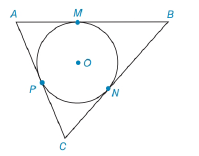Chapter 6.3, Problem 35EElementary Geometry For College St...

7th Edition
Alexander + 2 others
ISBN: 9781337614085

Solutions

Chapter
SectionElementary Geometry For College St...

7th Edition
Alexander + 2 others
ISBN: 9781337614085
Textbook Problem

Given: Tangents A B ¯ , B C ¯ ,     and     A C ¯     t o     ⊙ O at points M, N, and P, respectively. A B = 14 , B C = 16 , A C = 12 Find: AM, PC, and BNTo determine

To find:

To find AM, PC, and BN.

Explanation

Given that, Tangents AB¯,BC¯, and AC¯ to O at points M, N, and P, respectively AB=14,BC=16,AC=12

The tangents segments from an external point are congruent. Therefore,

AM=AP=xBM=BN=yCN=CP=z

The diagrammatic representation is given below,

We know that,

AB=AM+MB=x+yBC=BN+NC=y+zAC=AP+PC=x+z

Substitute the value of AB=14,BC=16,AC=12 in the above equation to get the following,

x+y=14...(1)y+z=16...(2)x+z=12...(3)

Solve equation (1) and (2) to get the following,

x+y+0z=140x+y+z=16()(

Still sussing out bartleby?

Check out a sample textbook solution.

See a sample solution

The Solution to Your Study Problems

Bartleby provides explanations to thousands of textbook problems written by our experts, many with advanced degrees!

Get Started

What is the lowest score in the following distribution?

Essentials of Statistics for The Behavioral Sciences (MindTap Course List)

Find all possible real solutions of each equation in Exercises 3144. x3x23x+3=0

Finite Mathematics and Applied Calculus (MindTap Course List)

Subtract and check: 75612397_

Elementary Technical Mathematics

Find the domain of the function. 32. f(x)=2x35x2+x6

Single Variable Calculus: Early Transcendentals, Volume I

True or False: f(x) is symmetric about the origin if f(x) =f(x).

Study Guide for Stewart's Single Variable Calculus: Early Transcendentals, 8th

The graph at the right has equation:

Study Guide for Stewart's Multivariable Calculus, 8th